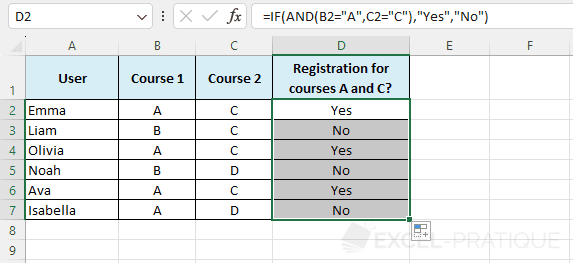# Excel Function: AND

The Excel function AND checks if all tests are TRUE and returns FALSE if one or more tests are FALSE.

This function is usually used in combination with other functions (as in the following example with the function IF).

Usage:

`=AND(test_1, test_2, etc)`

## Example of use

The goal here is to check if the user is registered for course A AND course C: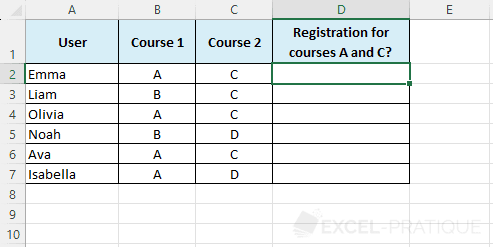Start by selecting the IF function and enter the Value_if_true and Value_if_false: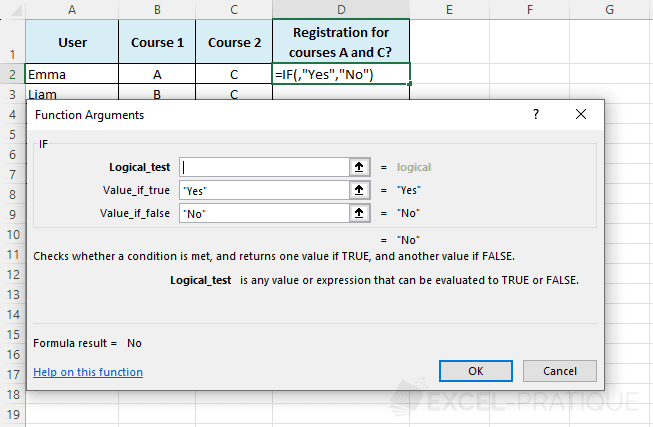Now click in Logical_test and select the AND function from the drop-down list: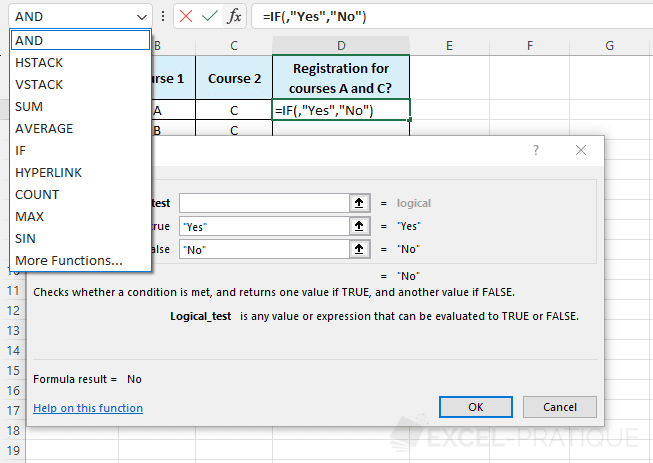Finally, enter the tests to be performed: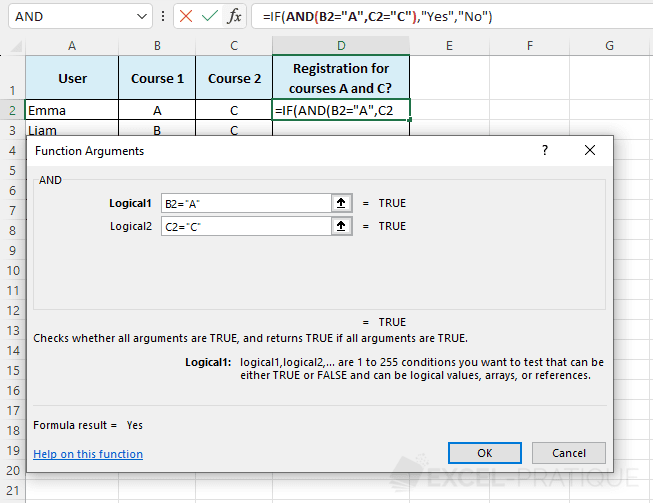The formula therefore returns "Yes" because all tests are TRUE:

``=IF(AND(B2="A",C2="C"),"Yes","No")``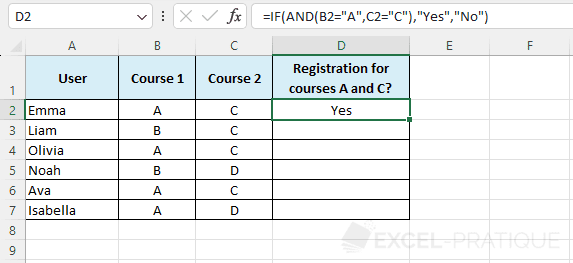Then stretch the formula to apply it to the other cells: